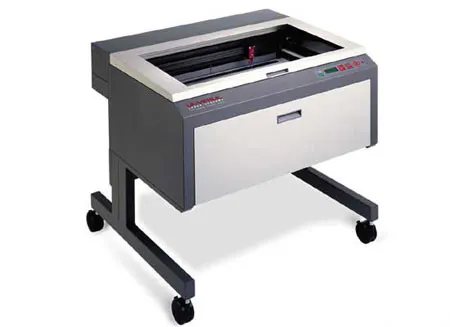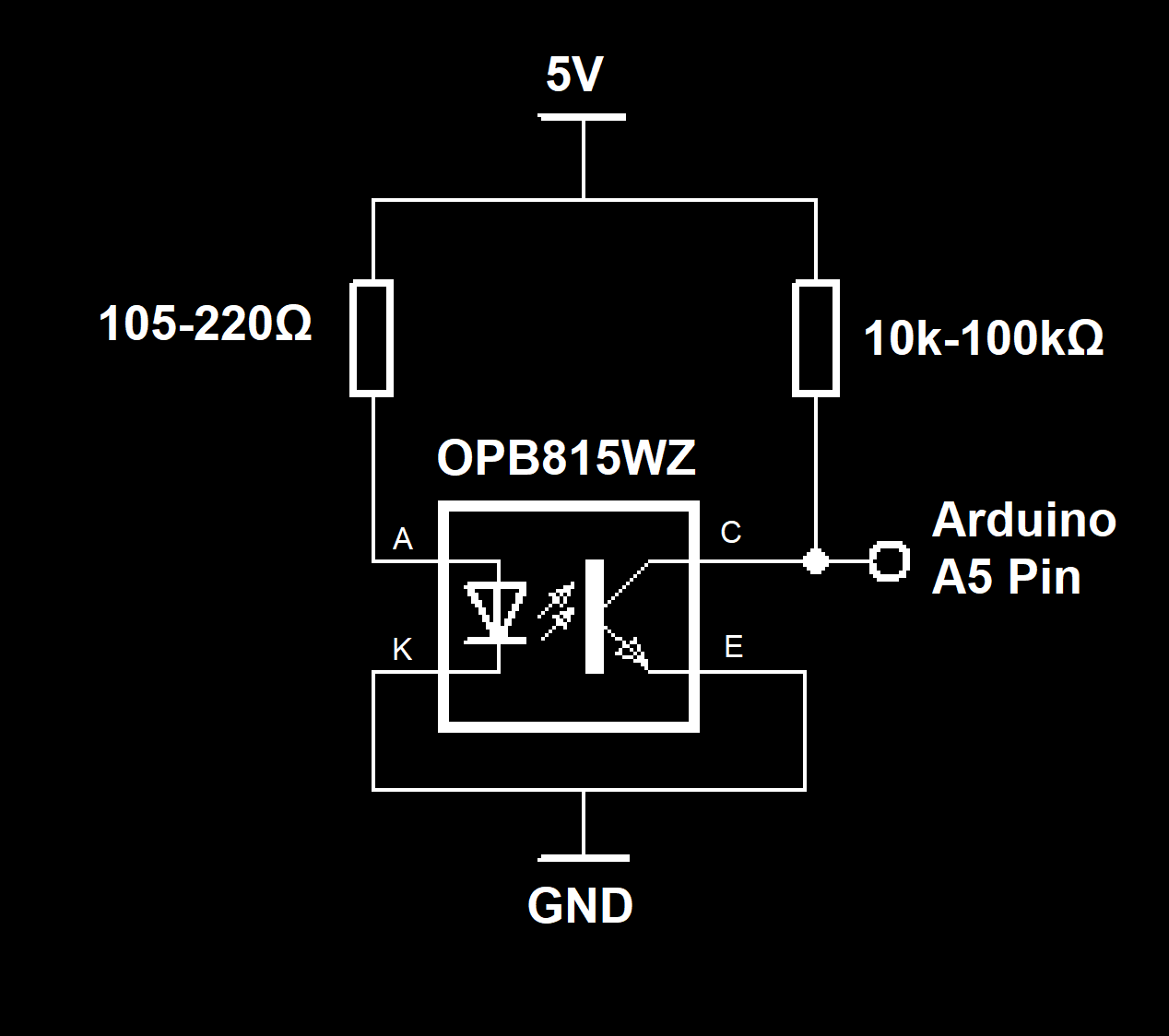Project showcase

Automated Pickup Winder Using an Arduino Uno © GPL3+

Project uses an Arduino Uno and a pair of stepper motors to wind tonewheel organ pickups.

• 5,116 views
• 11 respects

Components and suppliesArduino UNO
×1
 NEMA17 Stepper
×2
 LM8UU Bearing
×2
 8mmx150mm Rods
×2
 M3 Screws, Nuts, Heated Inserts
×20
 Laser-Cut Base Plate
×1
 Misc 3D Printed Parts
×6
 OPB815WZ Sensor
×1

Necessary tools and machines3D Printer (generic)Laser cutter (generic)

Apps and online services

Looking at what I wanted to do for a next project, I realized I have a lot of pickups I need to wind! This project came about mainly because of that.

Code

Arduino Uno SketchArduino
//    This program is free software: you can redistribute it and/or modify
//    the Free Software Foundation, either version 3 of the License, or
//    (at your option) any later version.
//
//    This program is distributed in the hope that it will be useful,
//    but WITHOUT ANY WARRANTY; without even the implied warranty of
//    MERCHANTABILITY or FITNESS FOR A PARTICULAR PURPOSE.  See the
//    GNU General Public License for more details.
//
//    You should have received a copy of the GNU General Public License
//    along with this program.  If not, see <https://www.gnu.org/licenses></https:>.

#define MAX_XPOS 64000  // Start of pickup
#define MIN_XPOS 15000  // End of pickup

#define ANALOGPIN A4
#define FLAGPIN A5

#define ENABLE 8
#define X_STEP 2
#define X_DIR 5
#define STEP 3
#define DIRECT 6
#define STEPLOOP 800

boolean toggle0 = 0;
boolean toggle1 = 0;
boolean toggle2 = 0;
boolean step1 = 0;
volatile int steps = 0;
volatile int turns = 0;
volatile boolean turnflag = 0;
bool flagVal = false;
bool xstepFlagHigh=false;
bool xstepFlagLow=true;

unsigned long xPos;
unsigned long timeA;
unsigned long timeMicros;
int xIncrement = 1;
unsigned int buffi = 0;

void setup(){
//setup will initialize serial, timers, and run a "homing" protocol

Serial.begin(115200);
Serial.write("begin");

//set pins as outputs
pinMode(ENABLE, OUTPUT);
pinMode(DIRECT, OUTPUT);
pinMode(STEP, OUTPUT);
pinMode(X_STEP,OUTPUT);
pinMode(X_DIR,OUTPUT);
pinMode(ANALOGPIN, INPUT);
pinMode(FLAGPIN, INPUT);
digitalWrite(ENABLE,LOW);
digitalWrite(DIRECT,LOW);

digitalWrite(X_DIR, HIGH);

long initSteps = 100000;

//move_forward to get out of any flags
for(int i = 0; i<3200; i++)
{
digitalWrite(X_STEP, HIGH);
delayMicroseconds(350);
digitalWrite(X_STEP,LOW);
delayMicroseconds(350);
}

//change direction and back into the flag
digitalWrite(X_DIR,LOW);
delay(500);

while(flagVal == false && initSteps>0)
{
digitalWrite(X_STEP, HIGH);
delayMicroseconds(350);
digitalWrite(X_STEP,LOW);
delayMicroseconds(350);
initSteps--;
}

xPos=0;

digitalWrite(X_DIR,HIGH);

//Move to start of pickup (MIN_XPOS)

for(int i = 0; i<MIN_XPOS; i++)
{
digitalWrite(X_STEP, HIGH);
delayMicroseconds(350);
digitalWrite(X_STEP,LOW);
delayMicroseconds(350);
xPos+=xIncrement;
}

digitalWrite(X_DIR,LOW);

cli();//stop interrupts

//Configure timer
TCCR1A = 0;
TCCR1B = 0;
TCNT1  = 0;
OCR1A = 24;
TCCR1B |= (1 << WGM12);
TCCR1B |= (1 << CS11) | (0 << CS10);
TIMSK1 |= (1 << OCIE1A);

sei();//allow interrupts

}//end setup

ISR(TIMER1_COMPA_vect)
//Timer ISR --> try to keep this uncomplicated
{
if (toggle1 && step1){
digitalWrite(3,HIGH);
toggle1 = 0;
}
else{
digitalWrite(3,LOW);
toggle1 = 1;
steps++;
}
if(steps>=3200)
{
if(step1)
{
turns++;
steps = 0;
turnflag = true;
}
}
}

//averages the array of past ADC values to length n
int average (int *readbuffer, unsigned int n)
{
int cumul = 0;
for(int i = 0; i<n; i++)
{

}

return (int)(cumul/n);
}

void loop(){

timeA = millis();
timeMicros = micros();

//deadzone between 950 and 1024 = "off".
//This means the spindle won't wind.

if(avg>=950)
{
step1=false;
}

else
{
step1 = true;
}

//Verify that it's the right time to step, verify no limit is flagged,
//and then take a step in the right direction

if(timeMicros%STEPLOOP <= STEPLOOP/2 && xstepFlagHigh == true)
{

if(flagVal==1 || xPos <= MIN_XPOS)
{
digitalWrite(X_DIR,HIGH);
xIncrement = 1;
}
else if(xPos >= MAX_XPOS)
{
digitalWrite(X_DIR,LOW);
xIncrement = -1;
}

digitalWrite(X_STEP,HIGH);
xPos+= xIncrement;

xstepFlagHigh = false;
xstepFlagLow = true;

}

//Toggle line low if scheduled
if(timeMicros%STEPLOOP > STEPLOOP/2 && xstepFlagLow == true)
{
digitalWrite(X_STEP,LOW);
xstepFlagLow = false;
xstepFlagHigh = true;
}

//Adjust speed of motor by tweaking timer interrupt

{
cli();
OCR1A = (int)(avg/4+36);
buffi = (buffi+1)%ARLEN;
sei();
}

{
}

//if a turn has completed, shoot out the total number of turns completed
if(turnflag)
{
turnflag = false;
Serial.print(turns);
Serial.print(" ");
Serial.print(flagVal);
Serial.print("\n");
}

}

Custom parts and enclosures

assembly_files_XSkovISRm4.zip

SchematicsPublished on

December 13, 2020

Members who respect this project

See similar projects
you might like

Arduino UNO Guitar Pedal

Project tutorial by electrosmash

• 91,825 views
• 241 respects

Turn an Arduino Uno into a MIDI Controller: Guitar Pedals

Project tutorial by Johan van Vugt

• 21,078 views
• 17 respects

by Romekmil

• 3,182 views
• 5 respects

Arduino Uno and Mozzi Drum Machine (+dancing head)

Project showcase by Roni Bandini

• 5,061 views
• 10 respects

Automated Film Developer

Project in progress by Tanner Stinson

• 2,684 views